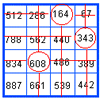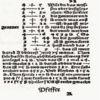#### You may also like### Marvellous Matrix

Follow the directions for circling numbers in the matrix. Add all the circled numbers together. Note your answer. Try again with a different starting number. What do you notice?### On What Day Did it Happen?

Read this article to find out the mathematical method for working out what day of the week each particular date fell on back as far as 1700.### Mathematical Symbols

A brief article written for pupils about mathematical symbols.

# Shapes on the Playground

### Why do this problem?

This problem is one that would be suitable for learners who are looking at measurement, regular polygons or revising the addition and subtraction of numbers to 100. It requires logical thinking and careful reading of the questions asked.

### Key questions

How long are each of the sides of every shape?
What is the perimeter of a hexagon/square/triangle/octagon?

What shapes does the problem say Ben drew?

### Possible extension

Learners could make a table of their results.

### Possible support

Suggest starting by drawing each the shapes and writing underneath how many metres the perimeter of each would be.